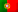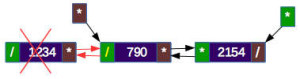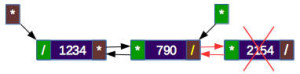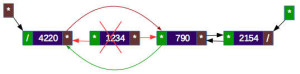Home > Technology > Computer Science > Programming > C++ > Doubly linked lists in C++ (Part II)

# Doubly linked lists in C++ (Part II)

In the first part of this article, I made an introduction to the doubly linked lists and I talked about methods to insert nodes. Let’s now see methods for removing nodes.### Removing at the tailThe removal at the tail is similar to the removal at the head but we use the pointer “tail” instead. In this case, we copy the pointer “previous” from the last node to the tail and then we can release it from memory. However, in this case we still have the pointer “next” of the “new last node” pointing to the node “2154” that is being deleted and shall be changed to NULL. We will see the code together with the removal at the middle of the list.

### Removing at the middle of the listTo remove a node at the middle of a double linked list, we can use two processes. We can search the node from the head and using the pointers “next“, or we can search the node from the tail and using the pointers “previous“.

In the first case, searching from the head, we have to search the node prior to the node we want to remove. Once we find it, and similar to a singly linked list, we copy the pointer “next” from the node we want to remove (node “1234” in the picture above) to the prior node (node “4220” in the picture), so it will now be pointing to the node that follows the node we want to remove (node “790” in the picture). Once again, and because we have a doubly linked list, we have to access the node “790” and change its pointer “previous” to the node prior to the node we want to remove. Having done this operations, the integrity of the list its guaranteed and we can release the node we want to remove from memory. In the singly linked lists, we saw that we could also use this process to remove a node at the tail, because by copying the pointer “next” from the last node (that shall be pointing to NULL) to the prior node, we are making this node the new last node. However, we need to pay attention when we are using doubly linked lists because we have the pointer “tail” and this one needs to be changed as well. Otherwise, it would be pointing to a node that no longer exists.

In the case we want to search the node we want to remove from the tail, the process will be similar but we will need to search the node that follows the node we want to remove and copy the pointer “previous” from the node we want to remove (“1234” in the picture) to the node “790”, so we have it pointing to the node prior to the one we want to remove (“4220”). Next, we need to access this node (“4220”) to change its pointer “next” to the node “790”. Finally, having done these operations, we can now release the node from memory. Still, in this case, if the node we were removing was the first node, by copying its pointer “previous” to the following node, we are making the following node the “new first node”. But, once again, we also need to change the pointer “head” to avoid having it pointing to a node that was removed.

Now, let’s see an example of a code to remove a node with a given value. In this example we will see how to search this node from the tail (from to end to the beginning).

```    int v = value_to_remove;
bool removed = false;
dnode = tail;
if (dnode->value==v) {
tail = dnode->previous;
DNode* precedent_dnode = dnode->previous;
if (precedent_dnode==NULL) {
}
else {
precedent_dnode->next = NULL;
}
free(dnode);
removed = true;
}
else {
while (dnode->previous!=NULL) {
DNode *remove = dnode->previous;
if (remove->value==v) {
dnode->previous = remove->previous;
DNode* precedent_dnode = remove->previous;
if (precedent_dnode==NULL) {
}
else {
precedent_dnode->next = remove->next;
}
free(remove);
removed = true;
break;
}
dnode = dnode->previous;
}
}
if (!removed) {
}
```

In the first line we declare a variable “v” with the value of the node we want to remove and, as we are searching the node from the tail, in line 3, we set the pointer “dnode”, used in the search, with the value of the pointer “tail“.

In line 4 we test if the value to remove is in the last node, pointed by the tail. If it is, we have a removal at the tail! So, in this case, we need to change the tail to point to the node prior to the node we want to remove (line 5) and get a pointer to that prior node so we can also update it (line 6). However, we also need to pay attention to a very important detail! And if we are removing the only node on the list? In this case, the pointer to the prior node is NULL (line 7) and the list will become empty, so we need to change the pointer “head” to NULL (line 8)! Regarding the pointer “tail”, as it receives the pointer “previous”, that is NULL, it will automatically become NULL. (1)
If it is not the only node on the list, we change the pointer “next” of the precedent node to NULL, since it will become to be the “new last node” (line 11).

If the value we want to remove is not in the last node, we follow with a “while” loop to search for it (line 17). Notice that, in line 18, we are going to search the value we want to remove in the node prior to the one used in the search, because we need to keep in “dnode” the reference to the node that follows the one we want to remove! When we find the node with the value we want to remove (line 19) we copy its pointer “previous” to the following node (line 20). In line 21, we will access to the node prior to the one we want to remove and we need to test if we are removing the first node! In this case, this precedent node will be NULL (line 22) and we have to change the head to the node that follows the one we want to remove (line 23) that will become the “new first node”! Also notice that, if the node we want to remove is the first node, it will have its pointer “previous” set to NULL and, when we made the copy in line 20, we will have the node that follows with the pointer “previous” set to NULL (first node / beginning of the list).

If the node we want to remove is not the first node, we copy its pointer “next” to its precedent node (line 26). The code will also test if the value we want to remove was found and displays a message if the value was not found.

Please note that, in this code, we didn’t test if the list was empty. But that test shall be done, as you will see in the complete code, down below.

Also, remember that in the end of the execution of the program, if the list is not empty, we will have to free all the nodes from memory. In the code of the singly linked list, I used a recursive method as an example. But we can use other solutions! For instance, we can successively delete the “first nodes” until the list becomes empty:

```    if (head!=NULL) {
cout << "Releasing node with the value: " << release->value << endl;
free(release);
}
tail = NULL;
}
```

Notice that it is not necessary to update the pointers “previous” or “next” because we are removing everything. Yet, after the loop ends, the pointer “head” will become NULL, but the pointer “tail” is still pointing to an allocation that no longer exists. So, after the loop, we set the pointer “tail” to NULL.

Let’s now see the complete code of this example:

```#include <iostream>
#include <stdlib.h>

using namespace std;

struct DNode {
int value;
DNode* next;
DNode* previous;
};

int main() {

DNode* dnode;
DNode* tail = NULL;

cout << "1 - Insert a value at the head" << endl;
cout << "2 - Insert a value at the tail" << endl;
cout << "3 - Remove node with a value x" << endl;
cout << "4 - List the node's values from the head" << endl;
cout << "5 - List the node's values from the tail" << endl;
cout << "0 - Exit program" << endl;

case '0':
break;
case '1':
cout << "Insert a new value for the beggining of the list: ";
dnode = (DNode*) malloc(sizeof(DNode));
dnode->previous = NULL;
dnode->next = NULL;
tail = dnode;
}
else {
}
break;
case '2':
char value_for_tail;
cout << "Insert a new value for the end of the list: ";
cin >> value_for_tail;
dnode = (DNode*) malloc(sizeof(DNode));
dnode->value=atoi(value_for_tail);
dnode->next = NULL;
if (tail==NULL) {
dnode->previous = NULL;
}
else {
tail->next = dnode;
dnode->previous = tail;
}
tail = dnode;
break;
case '3':
if (tail==NULL) {
cout << "The list is empty!" << endl;
}
else {
char value_to_remove;
cout << "Insert the value to remove: ";
cin >> value_to_remove;
int v = atoi(value_to_remove);
bool removed = false;
dnode = tail;
if (dnode->value==v) {
tail = dnode->previous;
DNode* precedent_dnode = dnode->previous;
if (precedent_dnode==NULL) {
}
else {
precedent_dnode->next = NULL;
}
free(dnode);
removed = true;
}
else {
while (dnode->previous!=NULL) {
DNode *remove = dnode->previous;
if (remove->value==v) {
dnode->previous = remove->previous;
DNode* precedent_dnode = remove->previous;
if (precedent_dnode==NULL) {
}
else {
precedent_dnode->next = remove->next;
}
free(remove);
removed = true;
break;
}
dnode = dnode->previous;
}
}
if (!removed) {
}
}
break;
case '4':
cout << "The list is empty!" << endl;
}
else {
while (dnode!=NULL) {
cout << "Value: " << dnode->value << endl;
dnode = dnode->next;
}
}
break;
case '5':
if (tail==NULL) {
cout << "The list is empty!" << endl;
}
else {
dnode = tail;
while (dnode!=NULL) {
cout << "Value: " << dnode->value << endl;
dnode = dnode->previous;
}
}
break;
default:
printf("Invalid option!");
break;
}

}

cout << "Releasing node with the value: " << release->value << endl;
free(release);
}
tail = NULL;
}

}```

In the third and last part of this article (under development) we will see an example of implementing a list with classes.

Follow us on the social networks and stay tuned with news from our multi-thematic blog – Out4Mind!

Footnote:

1. In the case of searching the node with the value we want to remove from the head, if it is the only node, the pointer “tail” will be set to NULL, but the pointer “head” won’t be! In this case, we need to change the pointer “head” to NULL, because the list will become empty and we want both pointers “head” and “tail” set to NULL.